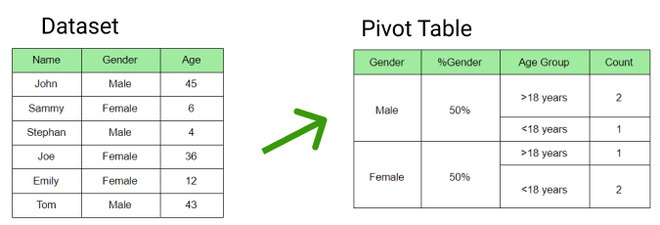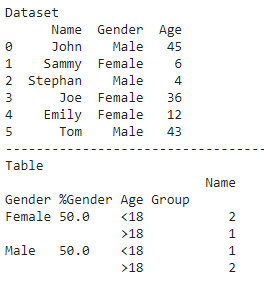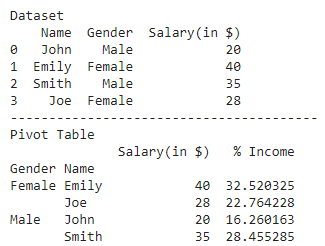How to include percentage in pivot table in Pandas?

• Last Updated : 24 Jan, 2021

Seaborn is an amazing visualization library for statistical graphics plotting in Python. It provides beautiful default styles and color palettes to make statistical plots more attractive. It is built on the top of matplotlib library and also closely integrated into the data structures from pandas.

Pivot table is used to summarize data which includes various statistical concepts. To calculate the percentage of a category in a pivot table we calculate the ratio of category count to the total count. Below are some examples which depict how to include percentage in a pivot table:

Example 1:

In the figure below, the pivot table has been created for the given dataset where the gender percentage has been calculated.Python3

 # importing pandas libraryimport pandas as pd  # creating dataframedf = pd.DataFrame({'Name': ['John', 'Sammy', 'Stephan', 'Joe', 'Emily', 'Tom'],                   'Gender': ['Male', 'Female', 'Male',                              'Female', 'Female', 'Male'],                   'Age': [45, 6, 4, 36, 12, 43]})print("Dataset")print(df)print("-"*40)  # categorizing in age groupsdef age_bucket(age):    if age <= 18:        return "<18"    else:        return ">18"  df['Age Group'] = df['Age'].apply(age_bucket)  # calculating gender percentagegender = pd.DataFrame(df.Gender.value_counts(normalize=True)*100).reset_index()gender.columns = ['Gender', '%Gender']df = pd.merge(left=df, right=gender, how='inner', on=['Gender'])  # creating pivot tabletable = pd.pivot_table(df, index=['Gender', '%Gender', 'Age Group'],                        values=['Name'], aggfunc={'Name': 'count',})  # display tableprint("Table")print(table)

Output:Example 2:

Here is another example which depicts how to calculate the percentage of a variable to its sum total in a particular column:

Python3

 # importing required librariesimport pandas as pdimport matplotlib.pyplot as plt  # creating dataframedf = pd.DataFrame({    'Name': ['John', 'Emily', 'Smith', 'Joe'],    'Gender': ['Male', 'Female', 'Male', 'Female'],    'Salary(in \$)': [20, 40, 35, 28]})  print("Dataset")print(df)print("-"*40)  # creating pivot tabletable = pd.pivot_table(df, index=['Gender', 'Name'])  # calculating percentagetable['% Income'] = (table['Salary(in \$)']/table['Salary(in \$)'].sum())*100  # display tableprint("Pivot Table")print(table)

Output:My Personal Notes arrow_drop_up